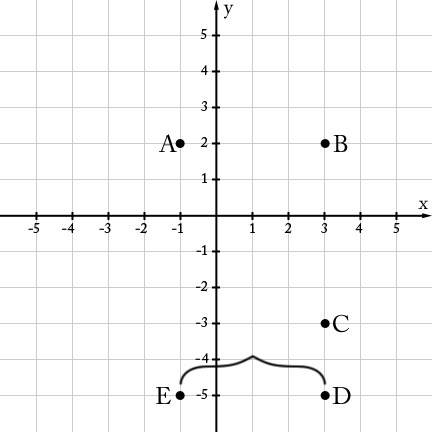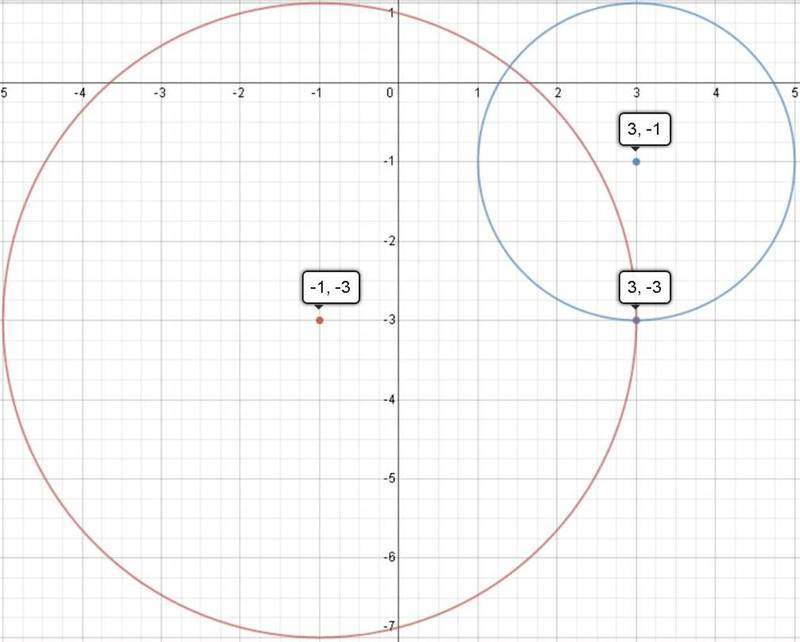# Distances between Points

Alignments to Content Standards: 6.NS.C.8

Some points are shown in the coordinate plane below.1. What is the distance between points B & C?
2. What is the distance between points D & B?
3. What is the distance between points D & E?
4. Which of the points shown above are $4$ units away from $(-1, -3)$ and $2$ units away from $(3, -1)$?

## IM Commentary

The purpose of this task is for students to solve a mathematical problem using points in the coordinate plane. This task also helps lay the foundation for understanding operations on signed numbers without formally introducing those notions, which are not expected until 7th grade (see 7.NS.1). Students will not have the full machinery needed to find distances between two arbitrary points in the plane until they have studied the Pythagorean Theorem in 8th grade. However, it is straightforward for them to find horizontal and vertical distances, which mirrors how one might represent sums and differences of signed numbers on a number line. The solution for the first part shows how these questions can be represented using absolute values (as described in standard 6.NS.C.8), although students are unlikely to make this connection without being asked to do so. If students are asked to solve this problem on their own first, it could be used to launch a discussion of the relationship between the the absolute value of coordinates of points in the plane and their distance to the respective axes.

Please note that students will have to assume that the points have integer coordinates, which is reasonable but worth mentioning.

## Solution

1. The distance between points B and C is 5 units, as indicated below.We can determine the distance between points $B$ and $C$ by counting the units between the two points since they are on the same vertical line which happens to be a grid line.

We can also note that the coordinates for $B$ are $(3,2)$ and so it is |2| units above the $x$-axis. Similarly, the coordinates for $C$ are $(3,-3)$, so it is |-3| units below the $x$-axis. Thus, the distance between $B$ and $C$ is $$|2|+|-3| = 5$$ units.

2. The distance between points $D$ and $B$ is 7 units, as indicated below.3. The distance between points $D$ and $E$ is 4 units, as indicated below.4. Point $C$ is the only one of points $A,…E$ that is both 4 units away from $(-1, -3)$ and 2 units away from $(3,-1)$. There is another point that satisfies these two criteria (which is the other intersection point of the circle of radius 4 and circle of radius 2 shown below), but it does not have integer coordinates and is not one of the points identified in the task statement.